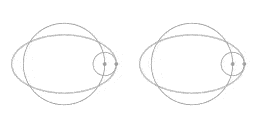# Coriolis Effect

Hi, everyone

We were told in class that the Coriolis Effect is due to the change in the radius in circular motion.

But an objtct moving with constant velocity on the edje of the circular system, like on the equator still experiencing Coriolis force, because the cross product 2wXv is not zero.

Why is that? There is no change in the radius, so what gives?

D H
Staff Emeritus

Doc Al
Mentor
Your instructor misspoke, or you misinterpreted what he said. In any case, you are correct: An object moving tangentially in a rotating frame will experience a Coriolis force, even though there is no radial motion.

O.K. It's a bit more clear now. But where does the Coriolis Effect come from then?

It is not a real force it is more like an imaginary force bcause of the frame of reference you have chosen .

Cleonis
Gold Member
O.K. It's a bit more clear now. But where does the Coriolis Effect come from then?

It's practical to first discuss two effects separately, and later combine them again.

- There is the case of tangential velocity (with respect to the rotating system), but no radial velocity. Then you get the effect that is the subject of the https://www.physicsforums.com/showthread.php?t=393932" that DH pointed out in an earlier message.

- There is the case of radial velocity, but no tangential velocity (initially).
In the case where the centripetal force is precisely enough to sustain the circumnavigating motion there is no radial velocity. To have some inward/outward radial velocity there needs to be a either a surplus of centripetal force, or not enough of it.The left hand side of the animation shows an object moving along an ellipse-shaped trajectory. A centripetal force sustains that trajectory, the animation depicts the case where the strength of the centripetal force is proportional to the distance to the central point.

Interestingly, the ellipse-shaped trajectory can be thought of as decomposed in a circle and an epi-circle.

The right hand side of the animation shows what the motion looks like as seen from a point of view that is rotating with constant angular velocity. As you can see the animation combines the cases of motion in radial direction and motion in tangential direction.

When a mass has a velocity with respect to the rotating system there is a tendency to deflect. This tendency to deflect is equally strong in all directions.

Last edited by a moderator:
D H
Staff Emeritus
O.K. It's a bit more clear now. But where does the Coriolis Effect come from then?
It comes from trying to use Newton's second law in a regime where Newton's second law isn't valid.

Let's use the merry-go-round example of the centrifugal force and Coriolis effect. You are on the merry-go-round and throw a ball to someone else on the merry-go-round. Someone else standing on the ground outside the merry-go-round is watching this affair.

The behavior of the ball as perceived by that outside observer is easy to explain. Once the ball has left your hand the only force acting on the ball is gravity. The ball follows a nice simple arc, pretty much the same as the arc that would have been followed if the ball was thrown by someone standing on the ground. In particular, the only curve is vertical. The horizontal component of the ball's trajectory follows a straight line. The relevant equation is good old F=ma.

The behavior of the ball as perceived by you is a bit harder to explain. The trajectory curves both vertically and horizontally. If you want to explain things via F=ma, there must be some horizontal component to the force. There is of course no real force that is curving the ball horizontally. The problem is trying to use F=ma where F=ma is not valid.

That said, there is nothing wrong with creating those fictitious forces to explain the ball's trajectory. It does work. It is important to always remember that those forces are not quite real. They are called fictitious forces for a good reason.

where does the Coriolis Effect come from then?

When no force acts upon an object and the object's motion is viewed from a non-inertial frame of reference, then the object appears to accelerate. In order to make this result consistent with F=ma, a fictitious force is postulated. For certain cases, this force is classified as a Coriolis force. (In other selected cases, the fictitious force is called a centrifugal force.) Relative to an inertial frame, these fictitious forces are not required, since Newton's 2nd law accurately governs the motion of an object relative to every inertial frame.

Thanks for your answers but it is just a bit fuzzy yet. Does anyone have a link to an article or some kind of resource about Coriolis?